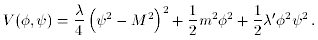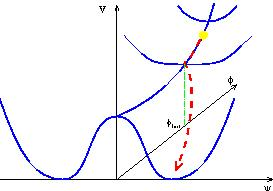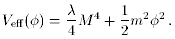5.7.2. Multi-field theories

A recent trend in inflationary model building has been the exploration of models with more than one scalar field. The classic example is the hybrid inflation model,  which seems particularly promising for particle physics model building. The simplest version has a potential with two fieldsandof the form(53)

which is illustrated in Figure 4. When2 is large, the minimum of the potential in the-direction is at= 0. The field rolls down this `channel' until it reachesinst2 =M2 /', at which point= 0 becomes unstable and the field rolls into one of the true minima at= 0 and= ±M.Figure 4. The potential for the hybrid inflation model. The field rolls down the channel at= 0 until it reaches the criticalvalue, then falls off the side to the true minimum at= 0 and= ±M.

While in the `channel', which is where all the interesting behaviour takes place, this is just like a single field model with an effective potential forof the form(54)

This is a fairly standard form, the unusual thing being the constant term, which would not normally be allowed as it would give a present-day cosmological constant. The most interesting regime is where that constant dominates, and it gives quite an unusual phenomenology. In particular, the energy density during inflation can be much lower than normal while still giving suitably large density perturbations, and secondly the fieldcan be rolling extremely slowly which is of benefit to particle physics model building.